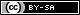# Dev Blog

 ./dev Original theme by orderedlist (CC-BY-SA) Where applicable, all content is licensed under a CC-BY-SA.# Arbitrary Binary Functions

Shannon's counting argument.

$$x \in \mathbb{B}^n \\ \\ f(X): \{0,1\}^n \mapsto \{0,1\}$$

There are $2^{2^n}$ different binary functions. Can we approximate this with less than $\frac{2^n}{c n}$ boolean gates for some constant $c$?

The strategy to show we can't is to overcount the number of boolean gates.

\begin{align} m & & \text{ boolean gates } (\frac{2^n}{c n}) \\ n & & \text{ inputs } \end{align}

Label each of the $m$ gates as either one of the $n$ inputs or one of the set of ${ \wedge, \vee, \neg }$. Assume a maximum of 2 inputs but that outputs can be traced to as many other gates as needed.

We have a total of $(n+3)$ labels for each gate and a maximum of $m^2$ input choices.

Each of the $m$ gates has a choice of label and choice of inputs, giving:

$$((n + 3) m^2)^m$$

$$\lg = \log_2$$

For $n$ large enough:

\begin{align} \lg( ((n + 3) m^2)^m ) & = \frac{2^n}{c n} [ \lg(n+3) + 2 n - 2 \lg(c n) ] \\ = \frac{2^n}{c} [ \frac{\lg(n+3)}{n} + 2 - \frac{2 \lg(c n)}{n} ] & < \frac{2^n}{c} [ \frac{2 \lg(n)}{n} + 2 - \frac{2 \lg(c n)}{n} ] \\ = \frac{2^n}{c} [ 2 + \frac{2}{n} ( \lg(n) - \lg(n) - \lg(c) ) ] & = \frac{2^n}{c} [ 2 - \frac{2}{n} \lg(c) ] \\ < 2 \frac{ 2^n }{ c } & = \frac{2^n}{ \frac{c}{2} } \end{align}

For $c>2$:

$$((n + 3) m^2)^m < 2^{2^n}$$

Even when we overcount, we still can't the count large enough to represent all binary functions.

src